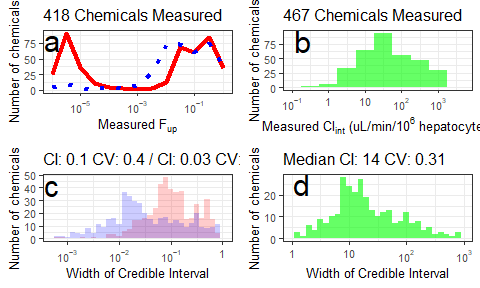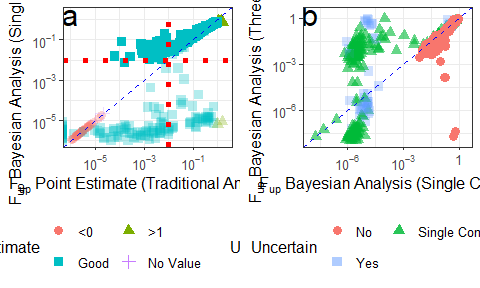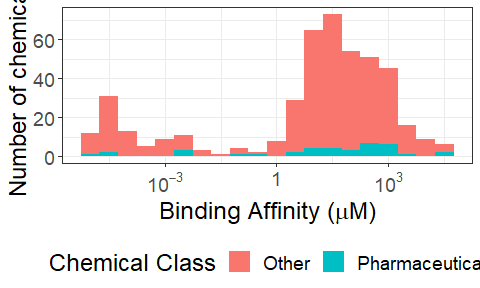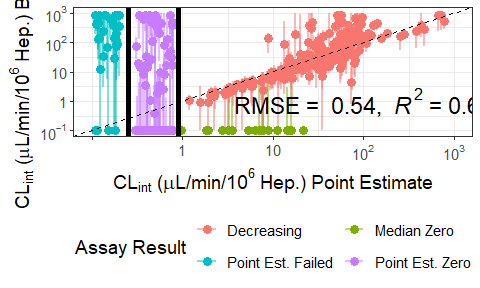Wambaugh et al. (2019): Creating Figures for the Manuscript

2021-05-07

This vignette provides the code used to generate the figures in Wambaugh et al. (2019) “Assessing Toxicokinetic Uncertainty and Variability in Risk Prioritization.”

John F Wambaugh, Barbara A Wetmore, Caroline L Ring, Chantel I Nicolas, Robert G Pearce, Gregory S Honda, Roger Dinallo, Derek Angus, Jon Gilbert, Teresa Sierra, Akshay Badrinarayanan, Bradley Snodgrass, Adam Brockman, Chris Strock, R Woodrow Setzer, Russell S Thomas

Toxicological Sciences, Volume 172, Issue 2, December 2019, Pages 235-251

https://doi.org/10.1093/toxsci/kfz205

Abstract

High(er) throughput toxicokinetics (HTTK) encompasses in vitro measures of key determinants of chemical toxicokinetics and reverse dosimetry approaches for in vitro-in vivo extrapolation (IVIVE). With HTTK, the bioactivity identified by any in vitro assay can be converted to human equivalent doses and compared with chemical intake estimates. Biological variability in HTTK has been previously considered, but the relative impact of measurement uncertainty has not. Bayesian methods were developed to provide chemical-specific uncertainty estimates for 2 in vitro toxicokinetic parameters: unbound fraction in plasma (fup) and intrinsic hepatic clearance (Clint). New experimental measurements of fup and Clint are reported for 418 and 467 chemicals, respectively. These data raise the HTTK chemical coverage of the ToxCast Phase I and II libraries to 57%. Although the standard protocol for Clint was followed, a revised protocol for fup measured unbound chemical at 10%, 30%, and 100% of physiologic plasma protein concentrations, allowing estimation of protein binding affinity. This protocol reduced the occurrence of chemicals with fup too low to measure from 44% to 9.1%. Uncertainty in fup was also reduced, with the median coefficient of variation dropping from 0.4 to 0.1. Monte Carlo simulation was used to propagate both measurement uncertainty and biological variability into IVIVE. The uncertainty propagation techniques used here also allow incorporation of other sources of uncertainty such as in silico predictors of HTTK parameters. These methods have the potential to inform risk-based prioritization based on the relationship between in vitro bioactivities and exposures.

##Prepate for session

library(data.table)
library(httk)
library(ggplot2)
library(gdata)
library(stringr)
library(scales)
library(grid)
library(reshape)
#>
#> Attaching package: 'reshape'
#> The following object is masked from 'package:dplyr':
#>
#>     rename
#> The following object is masked from 'package:cowplot':
#>
#>     stamp
#> The following object is masked from 'package:data.table':
#>
#>     melt

Define some needed code:

RMSE and R^2

This returns an expression with RMSE and R^2 for plotting:

Multiple plot function

From http://www.cookbook-r.com/Graphs/Multiple_graphs_on_one_page_(ggplot2)/ ggplot objects can be passed in …, or to plotlist (as a list of ggplot objects) - cols: Number of columns in layout - layout: A matrix specifying the layout. If present, ‘cols’ is ignored.

If the layout is something like matrix(c(1,2,3,3), nrow=2, byrow=TRUE), then plot 1 will go in the upper left, 2 will go in the upper right, and 3 will go all the way across the bottom.

Function to format scientific notation

This one leaves every other tickmark blank:

Figure 1:

Histograms depicting the median measured values (a, b) and 95% credible intervals (c, d) for fraction unbound in plasma (fup, a,c) and intrinsic hepatic clearance (Clint, b,d). In panel a, the distribution of estimated fup for the single protein concentration assay (solid line) is compared with the protein titration (three concentrations) method (dotted) line. In panel b, the median estimated Clint is shown. In panel c, the distribution with larger credible intervals corresponds to the single protein concentration assay, with a median credible interval is 0.1, corresponding to a precision of roughly +-0.05. When data from the protein titration are jointly analyzed (smaller credible intervals), the certainty is increased to a median credible interval of 0.029, or roughly +-0.015. The width of the credible interval is a measure of the certainty in an estimate, i.e., a smaller credible interval indicates greater certainty. For the fup values, a credible interval approaching 1 indicates that all possible values are consistent with the data, that is, the data do not help identify fup since fup must be between 0 and 1. In panel d the distribution of credible intervals for Clint is shown.

Fig1.table <- httk::wambaugh2019.raw
Fig1.table$Error1 <- Fig1.table$Base.Fup.High - Fig1.table$Base.Fup.Low Fig1.table$Error2 <- Fig1.table$Affinity.Fup.High - Fig1.table$Affinity.Fup.Low
Fig1.table$Sigma1 <- Fig1.table$Error1/(2*qnorm(0.975))
Fig1.table$Mean1 <- (Fig1.table$Base.Fup.High + Fig1.table$Base.Fup.Low)/(qnorm(0.975)) Fig1.table$CV1 <- Fig1.table$Sigma1/Fig1.table$Mean1
Fig1.table$Sigma2 <- Fig1.table$Error2/(2*qnorm(0.975))
Fig1.table$Mean2 <- (Fig1.table$Base.Fup.High + Fig1.table$Base.Fup.Low)/(qnorm(0.975)) Fig1.table$CV2 <- Fig1.table$Sigma2/Fig1.table$Mean2
Fig1.table$ErrorClint <- Fig1.table$CLint.1uM.High95th - Fig1.table$CLint.1uM.Low95th Fig1.table$SigmaClint <- Fig1.table$ErrorClint/(2*qnorm(0.975)) Fig1.table$MeanClint <- (Fig1.table$CLint.1uM.High95th + Fig1.table$CLint.1uM.Low95th)/(qnorm(0.975))
Fig1.table$CVClint <- Fig1.table$SigmaClint/Fig1.table$MeanClint Fupmeasured <- subset(Fig1.table,!is.na(Affinity.Fup.Med)) Fupmeasured <- subset(Fupmeasured,!duplicated(CAS)) CLintmeasured <- subset(Fig1.table,!is.na(CLint.1uM.Median)) CLintmeasured <- subset(CLintmeasured,!duplicated(CAS)) print(paste("New HTTK experimental measurements were made for",length(unique(Fig1.table$CAS)),"chemicals from the ToxCast HTS library."))
#>  "New HTTK experimental measurements were made for 496 chemicals from the ToxCast HTS library."

print(paste("Fup was successfully measured for",length(unique(subset(Fig1.table,!is.na(Affinity.Fup.Med))$CAS)),"chemicals from the ToxCast HTS library.")) #>  "Fup was successfully measured for 418 chemicals from the ToxCast HTS library." print(paste("CLint was successfully measured for",length(unique(subset(Fig1.table,!is.na(CLint.1uM.Median))$CAS)),"chemicals from the ToxCast HTS library."))
#>  "CLint was successfully measured for 467 chemicals from the ToxCast HTS library."

Fig1a <- ggplot(Fig1.table)+
geom_freqpoly(binwidth = 0.5,lwd=1.5,color="Red",aes(Base.Fup.Med))+
geom_freqpoly(binwidth = 0.5,lwd=1.5,lty=3,color="Blue",aes(Affinity.Fup.Med))+
xlab(expression(paste("Measured ",F[up]))) +
ylab("Number of chemicals")+
scale_x_log10(label=scientific_10,limits=c(10^-6,1.05))+
labs(title=paste(length(unique(subset(Fig1.table,!is.na(Affinity.Fup.Med))$CAS)),"Chemicals Measured"))+ theme_bw()+ theme( text = element_text(size=10)) + annotate("text", x=10^-6,y=80,size=8,label="a") print(paste("Median 100%:",signif(median(Fig1.table$Base.Fup.Med,na.rm=TRUE),2)))
#>  "Median 100%: 0.032"
print(paste("Median Titration:",signif(median(Fig1.table$Affinity.Fup.Med,na.rm=TRUE),2))) #>  "Median Titration: 0.028" Fig1b <- ggplot(Fig1.table)+ geom_histogram(binwidth = 0.5,alpha=0.6,fill="green",aes(CLint.1uM.Median))+ xlab(expression(paste("Measured ",Cl[int]," (uL/min/",10^6," hepatocytes)"))) + ylab("Number of chemicals")+ scale_x_log10(label=scientific_10,limits=c(10^-1,5*10^3))+ labs(title=paste(length(unique(subset(Fig1.table,!is.na(CLint.1uM.Median))$CAS)),"Chemicals Measured"))+
theme_bw()+
theme( text  = element_text(size=10)) +
annotate("text", x=2*10^-1,y=80,size=8,label="b")

print(paste(sum(Fig1.table$CLint.1uM.Median==0,na.rm=TRUE),"Zero Measurments")) #>  "142 Zero Measurments" print(paste("Median Non-Zero:",signif(median(Fig1.table$CLint.1uM.Median,na.rm=TRUE),3)))
#>  "Median Non-Zero: 16"

Fig1c <- ggplot(Fig1.table)+
geom_histogram(binwidth = 0.1,alpha=0.2,fill="Red",aes(Error1))+
geom_histogram(binwidth = 0.1,alpha=0.2,fill="Blue",aes(Error2))+
xlab("Width of Credible Interval") +
ylab("Number of chemicals")+
scale_x_log10(label=scientific_10,limits=c(5*10^-4,1.05))+
labs(title=paste("CI:",signif(median(Fig1.table$Error1,na.rm=TRUE),2),"CV:",signif(median(Fig1.table$CV1,na.rm=TRUE),1),"/ CI:",signif(median(Fig1.table$Error2,na.rm=TRUE),1),"CV:",signif(median(Fig1.table$CV2,na.rm=TRUE),1)))+
theme_bw()+
theme( text  = element_text(size=10))+
annotate("text", x=5*10^-4,y=42,size=8,label="c")

print(paste("Median 100% CI:",signif(median(Fig1.table$Error1,na.rm=TRUE),2),"CV:",signif(median(Fig1.table$CV1,na.rm=TRUE),2)))
#>  "Median 100% CI: 0.1 CV: 0.42"
print(paste("Median Titr. CI:",signif(median(Fig1.table$Error2,na.rm=TRUE),2),"CV:",signif(median(Fig1.table$CV2,na.rm=TRUE),2)))
#>  "Median Titr. CI: 0.029 CV: 0.1"

Fig1d <- ggplot(Fig1.table)+
geom_histogram(binwidth = 0.1,alpha=0.6,fill="green",aes(ErrorClint))+
xlab("Width of Credible Interval") +
ylab("Number of chemicals")+
scale_x_log10(label=scientific_10,limits=c(10^0,10^3))+
labs(title=paste("Median CI:",signif(median(Fig1.table$ErrorClint,na.rm=TRUE),2),"CV:",signif(median(Fig1.table$CVClint,na.rm=TRUE),2)))+
theme_bw()+
theme( text  = element_text(size=10))+
annotate("text", x=1.5,y=25,size=8,label="d")

print(paste("Median CI:",signif(median(Fig1.table$ErrorClint,na.rm=TRUE),2),"CV:",signif(median(Fig1.table$CVClint,na.rm=TRUE),2)))
#>  "Median CI: 14 CV: 0.31"

multiplot(Fig1a,Fig1c,Fig1b,Fig1d,cols=2,widths=c(1.75,1.75))
#> Warning: Removed 98 rows containing non-finite values (stat_bin).
#> Warning: Removed 148 rows containing non-finite values (stat_bin).
#> Warning: Removed 2 row(s) containing missing values (geom_path).

#> Warning: Removed 2 row(s) containing missing values (geom_path).
#> Warning: Removed 91 rows containing non-finite values (stat_bin).
#> Warning: Removed 85 rows containing non-finite values (stat_bin).
#> Warning: Removed 2 rows containing missing values (geom_bar).

#> Warning: Removed 2 rows containing missing values (geom_bar).
#> Warning: Transformation introduced infinite values in continuous x-axis
#> Warning: Removed 202 rows containing non-finite values (stat_bin).
#> Warning: Removed 2 rows containing missing values (geom_bar).
#> Warning: Transformation introduced infinite values in continuous x-axis
#> Warning: Removed 246 rows containing non-finite values (stat_bin).
#> Warning: Removed 2 rows containing missing values (geom_bar).#Figure 2: Distribution of Binding Affinities Employed in Bayesian fup Estimations. To jointly analyze the data from the RED assay conducted at three protein concentrations, a model for binding to protein with an dissociation constant (i.e., binding affinity) must be assumed. Although the specific binding protein (for example, hemoglobin) and the stoichiometry are unknown, this number provides a rough estimate for each chemical of how much that chemical would be expected to be impacted by the presence of plasma binding.

Fig2.table <- httk::wambaugh2019.raw
print(paste(sum(!is.na(Fig2.table$Fup.point)),"total successful Fup estimates using point estimation.")) #>  "437 total successful Fup estimates using point estimation." print(paste(sum(!is.na(Fig2.table$Base.Fup.Med)),"total successful Fup estimates using BAyesian analysis of top protein concentration."))
#>  "439 total successful Fup estimates using BAyesian analysis of top protein concentration."
Fig2.table$Error1 <- Fig2.table$Base.Fup.High - Fig2.table$Base.Fup.Low Fig2.table$Error2 <- Fig2.table$Affinity.Fup.High - Fig2.table$Affinity.Fup.Low
Fig2.table$Sigma1 <- Fig2.table$Error1/(2*qnorm(0.975))
Fig2.table$Mean1 <- (Fig2.table$Base.Fup.High + Fig2.table$Base.Fup.Low)/(qnorm(0.975)) Fig2.table$CV1 <- Fig2.table$Sigma1/Fig2.table$Mean1
Fig2.table$Sigma2 <- Fig2.table$Error2/(2*qnorm(0.975))
Fig2.table$Mean2 <- (Fig2.table$Base.Fup.High + Fig2.table$Base.Fup.Low)/(qnorm(0.975)) Fig2.table$CV2 <- Fig2.table$Sigma2/Fig2.table$Mean2
Fig2.table$Point.Estimate <- "Good" Fig2.table[Fig2.table$Fup.point<0&!is.na(Fig2.table$Fup.point),"Point.Estimate"]<-"<0" Fig2.table[Fig2.table$Fup.point>1&!is.na(Fig2.table$Fup.point),"Point.Estimate"]<-">1" Fig2.table[is.na(Fig2.table$Fup.point),"Point.Estimate"]<-"No Value"
Fig2.table[Fig2.table$Point.Estimate%in%c("<0","No Value"),"Fup.point"] <- Fig2.table[Fig2.table$Point.Estimate%in%c("<0","No Value"),"Base.Fup.Med"]
Fig2.table$Uncertain<-"Yes" Fig2.table[is.na(Fig2.table$CV1),"CV1"] <- -999
Fig2.table[Fig2.table$CV1<0.49,"Uncertain"] <- "No" Fig2.table[Fig2.table$CV1==-999,"CV1"] <- NA

Fig2a <- ggplot(subset(Fig2.table,!is.na(Base.Fup.Med)),aes(x=Fup.point,y=Base.Fup.Med,shape=Point.Estimate,alpha=Uncertain,colour=Point.Estimate)) +
geom_point(size=3)+
#         geom_errorbar(aes(ymax = Base.Fup.High, ymin=Base.Fup.Low))+
scale_y_log10(label=scientific_10,limits=c(10^-6,2)) +
scale_x_log10(label=scientific_10,limits=c(10^-6,2)) +
ylab(expression(paste(F[up]," Bayesian Analysis (Single Conc.)"))) +
xlab(expression(paste(F[up]," Point Estimate (Traditional Analysis)"))) +
geom_abline(intercept = 0, slope = 1,linetype="dashed", colour="Blue") +
#  geom_vline(xintercept = 1, size=2,linetype="dashed", colour="red")+
geom_vline(xintercept = 0.01, size=2,linetype="dotted", colour="red")+
geom_hline(yintercept = 0.01, size=2,linetype="dotted", colour="red")+
scale_colour_discrete(name="Point Estimate")+
scale_shape_discrete(name="Point Estimate") +
scale_alpha_manual(values=c(1,0.3))+
theme_bw() +
theme(legend.position="bottom", text  = element_text(size=12))+
annotate("text", size=8,x = 10^-6, y = 2, label = "a")+
guides(alpha=guide_legend(nrow=2,byrow=TRUE),colour=guide_legend(nrow=2,byrow=TRUE),shape=guide_legend(nrow=2,byrow=TRUE))

#print(Fig2a)

print(paste(sum(Fig2.table$Uncertain=="Yes"),"uncertain estimates using single conc.")) #>  "204 uncertain estimates using single conc." print(paste(sum(Fig2.table$Point.Estimate=="<0"),"chemicals with negative point estimates."))
#>  "40 chemicals with negative point estimates."
print(paste(sum(Fig2.table$Point.Estimate==">1"),"chemicals with Fup > 1.")) #>  "17 chemicals with Fup > 1." summary(lm(data=subset(Fig2.table,!is.na(Fup.point)&Fup.point>0&Base.Fup.Med>0),log(Fup.point)~log(Base.Fup.Med))) #> #> Call: #> lm(formula = log(Fup.point) ~ log(Base.Fup.Med), data = subset(Fig2.table, #> !is.na(Fup.point) & Fup.point > 0 & Base.Fup.Med > 0)) #> #> Residuals: #> Min 1Q Median 3Q Max #> -6.3128 -1.6260 0.1525 1.2047 11.2200 #> #> Coefficients: #> Estimate Std. Error t value Pr(>|t|) #> (Intercept) -1.33838 0.21342 -6.271 9.09e-10 *** #> log(Base.Fup.Med) 0.53667 0.02775 19.342 < 2e-16 *** #> --- #> Signif. codes: 0 '***' 0.001 '**' 0.01 '*' 0.05 '.' 0.1 ' ' 1 #> #> Residual standard error: 2.854 on 411 degrees of freedom #> Multiple R-squared: 0.4765, Adjusted R-squared: 0.4752 #> F-statistic: 374.1 on 1 and 411 DF, p-value: < 2.2e-16 Fig2.table$Uncertain2<-"Yes"
Fig2.table[is.na(Fig2.table$CV2),"CV2"] <- -999 Fig2.table[Fig2.table$CV2<0.49,"Uncertain2"] <- "No"
Fig2.table[Fig2.table$CV2==-999,"CV2"] <- NA Fig2.table$TopFail <- ""
Fig2.table[!is.na(Fig2.table$Affinity.Fup.Med)&Fig2.table$Uncertain2=="No","TopFail"]<-"No"
Fig2.table[Fig2.table$TopFail=="No"&!is.na(Fig2.table$Base.Fup.Med)&Fig2.table$Uncertain=="Yes","TopFail"]<-"Single Conc. Only" Fig2.table[!is.na(Fig2.table$Affinity.Fup.Med)&Fig2.table$Uncertain2=="Yes","TopFail"]<-"Yes" Fig2.table[!is.na(Fig2.table$Affinity.Fup.Med)&is.na(Fig2.table$Base.Fup.Med),"TopFail"] <- "Single Conc. Only" Fig2.table[is.na(Fig2.table$Base.Fup.Med),"Base.Fup.Med"] <-  Fig2.table[is.na(Fig2.table$Base.Fup.Med),"Affinity.Fup.Med"] Fig2b <- ggplot(subset(Fig2.table,!is.na(Affinity.Fup.Med)),aes(x=Base.Fup.Med,y=Affinity.Fup.Med,shape=TopFail,alpha=TopFail,colour=TopFail)) + geom_point(size=3)+ geom_point(data=subset(Fig2.table,TopFail=="No"),size=3)+ # geom_errorbar(aes(ymax = Fup.High.x, ymin=Fup.Low.x))+ # geom_errorbarh(aes(xmin=Fup.Low.y,xmax=Fup.High.y))+ scale_y_log10(label=scientific_10,limits=c(10^-8,2)) + scale_x_log10(label=scientific_10,limits=c(10^-8,2)) + xlab(expression(paste(F[up]," Bayesian Analysis (Single Conc.)"))) + ylab(expression(paste(F[up]," Bayesian Analysis (Three Conc.)"))) + geom_abline(intercept = 0, slope = 1,linetype="dashed", colour="Blue")+ scale_colour_discrete(name="Uncertain")+ scale_shape_discrete(name="Uncertain") + # annotate("text",size=8, x = 10^-4, y = 1, label = "B")+ scale_alpha_manual(name="Uncertain",values=c(1,0.6,0.3))+ theme_bw() + theme(legend.position="bottom", text = element_text(size=12))+ annotate("text", size=8,x = 10^-8, y = 2, label = "b")+ guides(alpha=guide_legend(nrow=2,byrow=TRUE),colour=guide_legend(nrow=2,byrow=TRUE),shape=guide_legend(nrow=2,byrow=TRUE)) #print(Fig2b) # Need to reduce to unique chemicals. Fupbaseworked <- subset(Fig2.table,!is.na(Base.Fup.Med)&Uncertain=="No") Fupbasenot <- subset(Fig2.table,!is.na(Base.Fup.Med)&Uncertain=="Yes") Fupbaseworked <- subset(Fupbaseworked,!duplicated(CAS)) Fupbasenot <- subset(Fupbasenot,!duplicated(CAS)) # Also need to count a chemical as a success if it worked for either duplicate: Fupbasenot <- subset(Fupbasenot,!(CAS%in%Fupbaseworked$CAS))

Fupaffinityworked <- subset(Fig2.table,!is.na(Affinity.Fup.Med)&Uncertain2=="No")
Fupaffinitynot <- subset(Fig2.table,!is.na(Affinity.Fup.Med)&Uncertain2=="Yes")
Fupaffinityworked <- subset(Fupaffinityworked,!duplicated(CAS))
Fupaffinitynot <- subset(Fupaffinitynot,!duplicated(CAS))
Fupaffinitynot <- subset(Fupaffinitynot,!(CAS%in%Fupaffinityworked$CAS)) print(paste(dim(Fupbaseworked),"total successful Fup estimates using single conc.")) #>  "234 total successful Fup estimates using single conc." print(paste(signif(dim(Fupbasenot)/(dim(Fupbaseworked)+dim(Fupbasenot))*100,3),"percent failure rate using single conc.")) #>  "44 percent failure rate using single conc." print(paste(dim(Fupaffinityworked),"total successful Fup estimates using protein titration.")) #>  "380 total successful Fup estimates using protein titration." print(paste(signif(dim(Fupaffinitynot)/(dim(Fupaffinityworked)+dim(Fupaffinitynot))*100,3),"percent failure rate using protein titration.")) #>  "9.09 percent failure rate using protein titration." print(paste(dim(Fupbasenot),"uncertain estimates using original protocol.")) #>  "184 uncertain estimates using original protocol." print(paste(dim(Fupaffinitynot),"uncertain estimates using protein titration.")) #>  "38 uncertain estimates using protein titration." print(paste(dim(Fupaffinityworked)-dim(Fupbaseworked),"chemicals that could not be measured at 100% plasma protein.")) #>  "146 chemicals that could not be measured at 100% plasma protein." Wetmore.data <- httk:::Wetmore.data W2015 <- subset(Wetmore.data,Reference=="Wetmore 2015") W2012 <- subset(Wetmore.data,Reference=="Wetmore 2012") print(paste("The Fup assay failed for ",signif(sum(W2012$Fub==0.005)/length(W2012$Fub)*100,3),"% of chemicals in {Wetmore, 2012} and ",signif(sum(W2015$Fub==0)/length(W2015$Fub)*100,3),"% of chemicals in {Wetmore, 2015}.")) #>  "The Fup assay failed for 38.6 % of chemicals in {Wetmore, 2012} and 11.5 % of chemicals in {Wetmore, 2015}." Fuplod <- subset(Fig2.table,TopFail=="Single Conc. Only") print(paste("Among the chemicals that were uncertain using the original protocol but could be measured using the new three concentration protocol, the median estimated Fup was ",signif(median(Fuplod$Affinity.Fup.Med),2),"with values as low as ",signif(min(Fuplod$Affinity.Fup.Med),2),"and as high as",signif(max(Fuplod$Affinity.Fup.Med),2),". Previously, a default of 0.005 has been assumed when Fup could not be measured {Rotroff, 2010}.}"))
#>  "Among the chemicals that were uncertain using the original protocol but could be measured using the new three concentration protocol, the median estimated Fup was  0.0041 with values as low as  9.5e-09 and as high as 0.95 . Previously, a default of 0.005 has been assumed when Fup could not be measured {Rotroff, 2010}.}"

summary(lm(data=subset(Fig2.table,!is.na(Base.Fup.Med)&Affinity.Fup.Med>0&Base.Fup.Med>0),log(Base.Fup.Med)~log(Affinity.Fup.Med)))
#>
#> Call:
#> lm(formula = log(Base.Fup.Med) ~ log(Affinity.Fup.Med), data = subset(Fig2.table,
#>     !is.na(Base.Fup.Med) & Affinity.Fup.Med > 0 & Base.Fup.Med >
#>         0))
#>
#> Residuals:
#>     Min      1Q  Median      3Q     Max
#> -8.8976 -0.4326  1.7346  2.2525 13.7985
#>
#> Coefficients:
#>                       Estimate Std. Error t value Pr(>|t|)
#> (Intercept)           -2.56126    0.24997  -10.25   <2e-16 ***
#> log(Affinity.Fup.Med)  0.67769    0.03246   20.88   <2e-16 ***
#> ---
#> Signif. codes:  0 '***' 0.001 '**' 0.01 '*' 0.05 '.' 0.1 ' ' 1
#>
#> Residual standard error: 3.735 on 445 degrees of freedom
#> Multiple R-squared:  0.4948, Adjusted R-squared:  0.4937
#> F-statistic: 435.8 on 1 and 445 DF,  p-value: < 2.2e-16

#dev.new(width=10,height=6)
multiplot(Fig2a,Fig2b,cols=2,widths=c(1.75,1.75))
#> Warning: Transformation introduced infinite values in continuous x-axis
#> Warning: Removed 12 rows containing missing values (geom_point).
#> Warning: Removed 1 rows containing missing values (geom_point).#Figure 3: Comparison of Bayesian fup and Uncertainty Estimations to Experimental Point Estimates. Two separate Bayesian analyses were performed: one including only those data collected at 100% PPP, and a second that used a binding model to relate data collected at 10%, 30%, and 100% PPP. In (A), fup point estimates <0 are plotted on the y-axis. These values are considered “good” if they lie between 0 and 1. The Bayesian median fup values (x-axis) derived from the 100% PPP data and the associated uncertainty estimates (vertical lines) were correlated with point estimates. Bayesian model estimates were constrained to be greater than zero and less than 1;. The red dotted line (at 1%) represents a previously assumed generic limit of quantification (Wetmore, et al., 2015; Wetmore, et al., 2012). In (B), the medians from the two Bayesian analyses are compared. In both plots, the diagonal dashed line indicates the identity line (“perfect predictions”). In both panels the Bayesian estimates are “uncertain” (semi-solid) if the CV was larger than 0.5 and certain (solid) otherwise.#Figure 4: Comparison of Bayesian Clint and Uncertainty Estimations to Experimental Point Estimates. Clint estimate for 1 uM chemical concentration displayed, though 1 and 10 uM were fit jointly. The size of the credible interval varies significantly from chemical to chemical, especially for the lower clearance rates. Plots of individual fits are provided as supplemental material .

clearance.table <- subset(httk::wambaugh2019.raw,!is.na(CLint.1uM.Median))

print(paste(sum(clearance.table$CLint.1uM.Median==0),"zero valued median Bayesian posteriors")) #>  "142 zero valued median Bayesian posteriors" print(paste(sum(clearance.table$CLint.1uM.Point==0&clearance.table$CLint.1uM.Median>0,na.rm=TRUE),"zero valued point estimates where median posterior>0")) #>  "43 zero valued point estimates where median posterior>0" print(paste(sum(clearance.table$CLint.1uM.Point>0&clearance.table$CLint.1uM.Median==0,na.rm=TRUE),"zero valued median Bayesian posteriors where point estimate was non-zero")) #>  "17 zero valued median Bayesian posteriors where point estimate was non-zero" print(paste(sum(is.na(clearance.table$CLint.1uM.Point)&clearance.table$CLint.1uM.Median==0,na.rm=TRUE),"zero valued median Bayesian posteriors where point estimate was NA")) #>  "5 zero valued median Bayesian posteriors where point estimate was NA" print(paste(sum(is.na(clearance.table$CLint.1uM.Point)&clearance.table$CLint.1uM.Median>0,na.rm=TRUE),"non-zero valued median Bayesian posteriors where point estimate was NA")) #>  "29 non-zero valued median Bayesian posteriors where point estimate was NA" clearance.table$Fit <- "Decreasing"
clearance.table[clearance.table$CLint.1uM.Median == 0,"Fit"] <- "Median Zero" #clearance.table[is.na(clearance.table$CLint.1uM.Point == 0),"Fit"] <- "Point Est. Missing"
for (i in 1:dim(clearance.table))
if (!is.na(clearance.table[i,"CLint.1uM.Point"]))
{
if (clearance.table[i,"CLint.1uM.Point"] == 0) clearance.table[i,"Fit"] <- "Point Est. Zero"
} else clearance.table[i,"Fit"] <- "Point Est. Failed"

set.seed(123456)
clearance.table[is.na(clearance.table$CLint.1uM.Point),"CLint.1uM.Point"]<-runif(sum(is.na(clearance.table$CLint.1uM.Point)),0.1,0.2)
clearance.table[clearance.table$CLint.1uM.Point==0,"CLint.1uM.Point"]<-runif(sum(clearance.table$CLint.1uM.Point==0),0.3,0.8)
clearance.table[clearance.table$CLint.1uM.Low95th==0,"CLint.1uM.Low95th"]<-0.1 clearance.table[clearance.table$CLint.1uM.Median==0,"CLint.1uM.Median"]<-0.1
clearance.table[clearance.table$CLint.1uM.High95th==0,"CLint.1uM.High95th"]<-0.1 clearance.table[clearance.table$CLint.1uM.High95th>1000,"CLint.1uM.High95th"]<-1000
clearance.table[clearance.table$CLint.1uM.Low95th<0.1,"CLint.1uM.Low95th"]<-0.1 clearance.fit <- lm(log10(CLint.1uM.Median)~log10(CLint.1uM.Point),data=subset(clearance.table,!is.na(CLint.1uM.Point)&CLint.1uM.Point>1)) Fig4 <- ggplot(clearance.table, aes(x=CLint.1uM.Point,y=CLint.1uM.Median,colour=Fit)) + geom_segment(aes(x=CLint.1uM.Point,xend=CLint.1uM.Point,y=CLint.1uM.Low95th,yend=CLint.1uM.High95th),size=1,alpha=0.5)+ geom_point(size=3) + scale_y_log10(label=scientific_10,limits=c(10^-1,1000)) + scale_x_log10(label=scientific_10_skip,limits=c(10^-1,1000)) + xlab(expression(paste(CL[int]," (",mu,"L/min/",10^{6}," Hep.) Point Estimate",sep="")))+ ylab(expression(paste(CL[int]," (",mu,"L/min/",10^{6}," Hep.) Bayesian",sep="")))+ geom_vline(xintercept = 0.25, size=2,colour="black")+ geom_vline(xintercept = 0.9, size=2,colour="black")+ geom_abline(intercept = 0, slope = 1,linetype="dashed")+ scale_colour_discrete(name="Assay Result")+ theme_bw() + theme(legend.position="bottom", text = element_text(size=14))+ guides(colour=guide_legend(nrow=2,byrow=TRUE))+ annotate("text",x = 10^2, y = 10^0,size=6, label = lm_R2(clearance.fit,prefix=""),parse=TRUE) #dev.new() print(Fig4) #> Warning in self$trans$transform(x): NaNs produced #> Warning: Transformation introduced infinite values in continuous x-axis #> Warning in self$trans$transform(x): NaNs produced #> Warning: Transformation introduced infinite values in continuous x-axis #> Warning in self$trans$transform(x): NaNs produced #> Warning: Transformation introduced infinite values in continuous x-axis #> Warning: Removed 18 rows containing missing values (geom_segment). #> Warning: Removed 19 rows containing missing values (geom_point).#Figure 5: Figure 5. Uncertainty Assessment for TK Quantities half-life and Css. The impact of uncertainty on estimated values for fup and Clint could in turn affect additional TK quantities, for example elimination half-life (t1/2, shown in panel A) and steady-state serum concentration (Css, shown in panel B). In both plots, the x-axis indicates the value that would have been estimated with previous methods, while the y-axis indicates the median and 95% credible interval for values calculated with our Bayesian method. Chemicals plotted with triangles are those that could be estimated with the previous, point estimate methods, while chemicals plotted with circles could not. The dashed line indicates the identity (perfect predictor) line. Chemicals that had no point estimate are plotted on the identity line. # This section takes a long time because calc_vdist is not yet data.table compatible: ceetox <- as.data.table(subset(httk::wambaugh2019,!is.na(Human.Funbound.plasma)&!is.na(Human.Clint)&!is.na(logP))) httk_cas <- ceetox$CAS[ceetox$CAS %in% get_cheminfo(model="3compartmentss")] # back up chem.physical_and_invtro.data: HTTK.data <- chem.physical_and_invitro.data # Use the point estimates: chem.physical_and_invitro.data <- add_chemtable(httk::wambaugh2019.raw,current.table=chem.physical_and_invitro.data,data.list=list(Compound="Name",CAS="CAS",Clint="CLint.1uM.Point",Funbound.plasma="Fup.point"),reference="Wambaugh 2019",species="Human",overwrite=TRUE) schmitt_cas <- httk_cas[httk_cas %in% get_cheminfo(model="schmitt")] ceetox[CAS%in%schmitt_cas, hlpoint:=log(2)/httk::calc_elimination_rate(chem.cas=CAS), by=.(CAS)] chem.physical_and_invitro.data <- HTTK.data ceetox[CAS%in%schmitt_cas, hl_975:=hlfun_u(chemcas=CAS, MW=MW, probs=0.975), by=.(CAS)] ceetox[CAS%in%schmitt_cas, hl_med:=hlfun_u(chemcas=CAS, MW=MW, probs=0.5), by=.(CAS)] ceetox[CAS%in%schmitt_cas, hl_25:=hlfun_u(chemcas=CAS, MW=MW, probs=0.025), by=.(CAS)] ceetox[,hlpointEstimated:=TRUE] ceetox[is.na(hlpoint),hlpointEstimated:=FALSE] ceetox[is.na(hlpoint),hlpoint:=hl_med] hlfit <- lm(data=subset(ceetox,hlpointEstimated),log10(hlpoint)~log10(hl_med)) Fig5a <- ggplot(data=subset(ceetox,hlpointEstimated),aes(x=hlpoint,y=hl_med)) + geom_point(size=3,alpha=0.5) + geom_errorbar(aes(ymax = hl_975, ymin=hl_25),alpha=0.3)+ scale_y_log10(label=scientific_10,limits=c(1,10^6)) + scale_x_log10(label=scientific_10,limits=c(1,10^6)) + xlab("half-life (h) Point Estimate")+ ylab("Bayesian half-life (h)")+ geom_abline(intercept = 0, slope = 1,linetype="dashed",color="blue")+ annotate("text", size=8,x = 1, y = 10^6, label = "A")+ theme_bw() + theme(text = element_text(size=18))+ annotate("text",x = 3*10^3, y = 10^0,size=5, label = lm_R2(hlfit,prefix=""),parse=TRUE) cssfit <- lm(data=ceetox,log10(cssmed)~log10(cssmed_u)) Fig5b <- ggplot(data=ceetox,aes(x=cssmed,y=cssmed_u)) + geom_point(size=3,alpha=0.5) + geom_errorbar(aes(ymin=css25_u,ymax=css975_u),alpha=0.3)+ scale_y_log10(label=scientific_10,limits=c(10^-3,10^5)) + scale_x_log10(label=scientific_10,limits=c(10^-3,10^5)) + xlab(expression(paste(C[ss]," Point Estimate (uM)")))+ ylab(expression(paste("Bayesian ",C[ss]," (uM)")))+ annotate("text", size=8,x = 10^-3, y = 10^5, label = "B")+ theme_bw() + theme(text = element_text(size=18)) + geom_abline(intercept = 0, slope = 1,linetype="dashed",color="blue")+ annotate("text",x = 10^2, y = 10^-3,size=5, label = lm_R2(cssfit,prefix=""),parse=TRUE) #dev.new(width=8,height=5) multiplot(Fig5a,Fig5b,cols=2,widths=c(1.75,1.75)) #Figure 6: Relative contributions of uncertainty and variability to differences between the 95th percentile and median Css. Monte Carlo analysis of both variability (triangles) and uncertainty (circles) give distributions that can be characterized by a 95th percentile Css for which individuals achieve a higher Css for the same fixed dose rate – these individuals can be considered more “sensitive” to chemical exposure. Uncertainty and variability can be combined (squares) to estimate a 95th percentile reflecting both factors. For most chemicals, variability contributes more than uncertainty to the difference between the Css predicted for the median Clint and fup values. set.seed(42) ceetox[CAS%in%httk_cas, css95_uv:=calc_mc_css(chem.cas=CAS, clint.pvalue.threshold=1, #Don't need to use p-values here (Bayesian) output.units="uM", model="3compartmentss", species="Human"), by=.(CAS)] set.seed(42) ceetox[CAS%in%httk_cas, css95_v:=calc_mc_css(chem.cas=CAS, clint.pvalue.threshold=1, #Don't need to use p-values here (Bayesian) output.units="uM", clint.meas.cv=NULL, fup.meas.cv=NULL, clint.pop.cv=0.3, fup.pop.cv=0.3, model="3compartmentss", species="Human"), by=.(CAS)] set.seed(42) ceetox[CAS%in%httk_cas, css95_u:=cssfun_u(chemcas=CAS, MW=MW), by=.(CAS)] set.seed(42) ceetox[CAS%in%httk_cas, cssmed_u:=cssfun_u(chemcas=CAS, MW=MW, probs=0.5), by=.(CAS)] set.seed(42) ceetox[CAS%in%httk_cas, css25_u:=cssfun_u(chemcas=CAS, MW=MW, probs=0.025), by=.(CAS)] set.seed(42) ceetox[CAS%in%httk_cas, css975_u:=cssfun_u(chemcas=CAS, MW=MW, probs=0.975), by=.(CAS)] ceetox[CAS%in%httk_cas, cssmed:=httk::calc_analytic_css(chem.cas=CAS, clint.pvalue.threshold=1, output.units="uM", model="3compartmentss", species="Human", suppress.messages=TRUE), by=.(CAS)] # Go back to Bayes estimates: chem.physical_and_invitro.data <- HTTK.data dt_melt <- data.table::melt(ceetox[, .(Compound, CAS, css95_u, css95_v, css95_uv, cssmed)], id.vars=c("Compound","CAS", "cssmed"), variable.name="type", value.name="css95") dt_melt[, type:=gsub(x=type, pattern="css95_uv", replacement="Both", fixed=TRUE)] dt_melt[, type:=gsub(x=type, pattern="css95_u", replacement="Uncertainty", fixed=TRUE)] dt_melt[, type:=gsub(x=type, pattern="css95_v", replacement="Variability", fixed=TRUE)] #now set the factor levels explicitly dt_melt[, type:=factor(type, levels=c("Uncertainty", "Variability", "Both"))] dt_melt[, Norm:=css95/cssmed] #now set the factor levels explicitly dt_melt[, Compound:=factor(Compound, levels=dt_melt[type=="Both",Compound][order(dt_melt[type=="Both",Norm])])] Fig6 <- ggplot(data=dt_melt) + geom_point(size=2,alpha=0.7,aes(x=Compound, y=Norm, color=type, shape=type)) + scale_y_log10(label=scientific_10,limits=c(0.9,500)) + ylab(expression(paste("Ratio of ",C[ss]," ",95^th," Percentile to Median Estimate"))) + xlab("Chemicals")+ scale_colour_discrete(name=expression(paste(C[ss]," Varied to Reflect")))+ scale_shape_discrete(name=expression(paste(C[ss]," Varied to Reflect"))) + theme_bw() + theme(legend.position="bottom",axis.text.x = element_blank())+ annotate("text", size=8,x = levels(dt_melt$Compound)[length(unique(dt_melt$Compound))/2], y = 400, label = paste("Median Ratio for Uncertainty:",signif(median(subset(dt_melt,type=="Uncertainty")$Norm),3)))+
annotate("text", size=8,x = levels(dt_melt$Compound)[length(unique(dt_melt$Compound))/2], y = 200, label = paste("Median Ratio for Variability:",signif(median(subset(dt_melt,type=="Variability")$Norm),3)))+ annotate("text", size=8,x = levels(dt_melt$Compound)[length(unique(dt_melt$Compound))/2], y = 100, label = paste("Median Ratio for Both:",signif(median(subset(dt_melt,type=="Both")$Norm),3)))

#dev.new(width=10,height=6)
print(Fig6)

#Figure 7: High throughput risk-based prioritization can be conducted by using doses predicted to cause bioactivity identified by high throughput screening. High throughput exposure estimates have large uncertainty, indicated by the triangles (median of distribution) and vertical bar (upper 95th percentile range). There are many in vitro activities identified for each chemical, the 80% interval of equivalent dose rates is indicated by the vertical red bar, with a horizontal slash indicating the median and the circle plot points indicating the 10th percentile of the bioactive concentration. Anywhere the entire exposure bar is below the bioactivity circle there is 95% probability that the median exposure will not cause a dose sufficient to cause the 10th percentile in vitro bioactivity. Chemicals are sorted from left-to-right by the margin between the 10th percentile bioactivity (small circle) and the upper 95th percentile limit on estimated median intake rate. In panel A, all chemicals for which Css could be calculated are shown using only variability. In panel B, the shaded region of panel A is shown, using both variability and uncertainty to calculate the bioactive dose. Arrows in panel B identify six chemicals where exposure and 10th percentile bioactivity potentially overlap that would have been missed as priority chemicals if uncertainty analysis was not performed. Two overlapping bars are shown for each chemical’’s bioactive doses – the upper one is for variability alone (as in Panel A), the lower is for uncertainty and variability together.

# Head to ftp://newftp.epa.gov/COMPTOX/High_Throughput_Screening_Data/Previous_Data/ToxCast_Data_Release_Oct_2015/ to get the ToxCast/Tox21 data:
# These are the concentrations that caused activity in exess of the background (ACC) "hits".
#
# # Load the ACC table into an object Tox21.acc:
# # Subset to the chemicals of interest:
# Tox21.acc <- subset(Tox21.acc,casn%in%Fig1.table$CAS) # # We only need hits: # Tox21.acc <- subset(Tox21.acc,hitc==1) # # # Pare this down to just the data we need: # Tox21.acc <- Tox21.acc[,c("code","chid","chnm","casn","aenm","modl_acc","flags")] # # # In this vignette we use the precalculated quantiles of the ACC's # # to help keep the HTTK package smaller: # # wambaugh2019.tox21 <- NULL # for (this.chem in sort(unique(Tox21.acc$casn)))
# {
#   this.subset <- subset(Tox21.acc,casn==this.chem)
#   this.row <- data.frame(casn=this.chem,
#     med.conc =
#       median(10^(this.subset$modl_acc)), # q10.conc = # quantile(10^(this.subset$modl_acc),0.1),
#     low.conc =
#       min(10^(this.subset$modl_acc)), # q90.conc = # quantile(10^(this.subset$modl_acc),0.9),
#     high.conc =
#       max(10^(this.subset$modl_acc)), # stringsAsFactors = F) # wambaugh2019.tox21 <- rbind(wambaugh2019.tox21, this.row) # } chem.preds <- httk::wambaugh2019.seem3 directnhanes.preds <- httk::wambaugh2019.nhanes Tox21.acc.numeric <- httk::wambaugh2019.tox21 #Subset to those chemicals with HTS hits: human.hits <- subset(ceetox,CAS%in%Tox21.acc.numeric$casn)

# Now calculate the oral equivalent dose for each chemical:
# Must make sure that CSS is in units of uM!!
for (this.chem in human.hits$CAS) { med.conc <- Tox21.acc.numeric[Tox21.acc.numeric$casn==this.chem,"med.conc"]
q10.conc <-
Tox21.acc.numeric[Tox21.acc.numeric$casn==this.chem,"q10.conc"] low.conc <- Tox21.acc.numeric[Tox21.acc.numeric$casn==this.chem,"low.conc"]
q90.conc <-
Tox21.acc.numeric[Tox21.acc.numeric$casn==this.chem,"q90.conc"] high.conc <- Tox21.acc.numeric[Tox21.acc.numeric$casn==this.chem,"high.conc"]
css95.v <- human.hits[human.hits$CAS==this.chem][["css95_v"]] css95.uv <- human.hits[human.hits$CAS==this.chem][["css95_uv"]]
human.hits[human.hits$CAS==this.chem,"HTS.Median.ACC"] <- med.conc human.hits[human.hits$CAS==this.chem,"HTS.Q10.ACC"] <- q10.conc
human.hits[human.hits$CAS==this.chem,"HTS.Q90.ACC"] <- q90.conc human.hits[human.hits$CAS==this.chem,"HTS.Low.ACC"] <- low.conc
human.hits[human.hits$CAS==this.chem,"HTS.High.ACC"] <- high.conc human.hits[human.hits$CAS==this.chem,"HTS.Median.equivdose.v"] <- med.conc/css95.v
human.hits[human.hits$CAS==this.chem,"HTS.Q10.equivdose.v"] <- q10.conc/css95.v human.hits[human.hits$CAS==this.chem,"HTS.Q90.equivdose.v"] <- q90.conc/css95.v
human.hits[human.hits$CAS==this.chem,"HTS.Low.equivdose.v"] <- low.conc/css95.v human.hits[human.hits$CAS==this.chem,"HTS.High.equivdose.v"] <- high.conc/css95.v
human.hits[human.hits$CAS==this.chem,"HTS.Median.equivdose.uv"] <- med.conc/css95.uv human.hits[human.hits$CAS==this.chem,"HTS.Q10.equivdose.uv"] <- q10.conc/css95.uv
human.hits[human.hits$CAS==this.chem,"HTS.Q90.equivdose.uv"] <- q90.conc/css95.uv human.hits[human.hits$CAS==this.chem,"HTS.Low.equivdose.uv"] <- low.conc/css95.uv
human.hits[human.hits$CAS==this.chem,"HTS.High.equivdose.uv"] <- high.conc/css95.uv } #Add the exposure predictions: for (this.chem in human.hits$CAS)
{
print(this.chem)
# If we have direct inferrences from NHANES, use those exposures:
if (this.chem %in% directnhanes.preds$CASRN) { human.hits[human.hits$CAS==this.chem,"Exposure.median"] <- directnhanes.preds[directnhanes.preds$CASRN==this.chem,"lP"] human.hits[human.hits$CAS==this.chem,"Exposure.high"] <- directnhanes.preds[directnhanes.preds$CASRN==this.chem,"lP.max"] # Otherwise use the heuristics model (Wambaugh 2014): } else if (this.chem %in% chem.preds$CAS) {
human.hits[human.hits$CAS==this.chem,"Exposure.median"] <- chem.preds[chem.preds$CAS==this.chem,"seem3"]
human.hits[human.hits$CAS==this.chem,"Exposure.high"] <- chem.preds[chem.preds$CAS==this.chem,"seem3.u95"]
}
}

# Drop chemicals without exposure predictions:
human.hits<- subset(human.hits,!is.na(Exposure.median))

# This code puts the chemicals into order by margine between exposure and activity:
chem.names <- unique(human.hits$Compound) potency <- rep(Inf,times=length(chem.names)) names(potency) <- chem.names potency.u <- potency for (this.chem in chem.names) { this.subset <- subset(human.hits,Compound==this.chem) potency[this.chem] <- this.subset$HTS.Q10.equivdose.v/this.subset$Exposure.high potency.u[this.chem] <- this.subset$HTS.Q10.equivdose.uv/this.subset$Exposure.high } potency <- sort(potency) human.hits$Compound <- factor(human.hits\$Compound, levels=names(potency))

potency.u <- potency.u[names(potency.u)[potency.u<1]]
potency.u <- potency.u[names(potency)[potency>1]]

new.overlaps <- names(potency.u[!is.na(potency.u)])
first.change <- new.overlaps
last.change <- new.overlaps[length(new.overlaps)]
window.start <- names(potency)[which(names(potency)==first.change)-5]
window.end <- names(potency)[which(names(potency)==last.change)+5]
window.names <- names(potency)[(which(names(potency)==first.change)-5):(which(names(potency)==last.change)+5)]
# Initialize a new plot:
Fig7a <- ggplot(data=human.hits) +
geom_segment(aes(x=Compound,xend=Compound,y=HTS.Q10.equivdose.v,yend=HTS.Q90.equivdose.v),size=1,color="white",alpha=0.5) +
geom_rect(mapping=aes(xmin=window.start,xmax=window.end,ymin=10^-9,ymax=10^3),color="grey",alpha=0.5)+
geom_segment(aes(x=Compound,xend=Compound,y=HTS.Q10.equivdose.v,yend=HTS.Q90.equivdose.v),size=1,color="red",alpha=0.5) +
geom_point(aes(x=Compound,y=HTS.Median.equivdose.v),shape=3) +
geom_point(aes(x=Compound,y=HTS.Q10.equivdose.v)) +
theme_bw() +
theme(axis.text.x = element_blank()) +
theme(axis.title.x = element_text(size=16)) +
theme(axis.title.y = element_text(size=16)) +
scale_y_log10(label=scientific_10,limits = c(10^-9,10^3)) +
geom_segment(aes(x=Compound,xend=Compound,y=Exposure.high,yend=Exposure.median),size=1,color="blue",alpha=0.5) +
geom_point(aes(x=Compound,y=Exposure.median),shape=2) +
ylab("Bioactive Dose & Exposure\nmg/kg BW/day\n(Variability Only)") +
xlab("Chemicals Ranked By Ratio Between Bioactive Dose and Exposure")+
annotate("text", size=8,x = names(potency), y = 100, label = "a")

# Initialize a new plot:
Fig7c <- ggplot() +
geom_segment(data=human.hits,aes(x=Compound,xend=Compound,y=HTS.Q10.equivdose.v,yend=HTS.Q90.equivdose.v),size=3,color="grey") +
geom_segment(data=human.hits,aes(x=Compound,xend=Compound,y=HTS.Q10.equivdose.uv,yend=HTS.Q90.equivdose.uv),size=3,color="red",alpha=0.5) +
geom_point(data=human.hits,aes(x=Compound,y=HTS.Median.equivdose.uv),shape=3,size=2) +
geom_point(data=human.hits,aes(x=Compound,y=HTS.Q10.equivdose.uv),size=2) +
theme_bw() +
theme(axis.text.x = element_text(angle = 60, hjust = 1,size=6)) +
theme(axis.text.x = element_blank()) +
theme(axis.title.x = element_text(size=16)) +
theme(axis.title.y = element_text(size=16)) +
xlim(window.names) +
scale_y_log10(label=scientific_10,limits = c(10^-8,2*10^2)) +
geom_segment(data=human.hits,aes(x=Compound,xend=Compound,y=Exposure.high,yend=Exposure.median),size=3,color="blue",alpha=0.5) +
geom_segment(data=subset(human.hits,Compound%in%names(potency.u)),aes(x=Compound,xend=Compound,y=10^-8,yend=Exposure.median/1.5),size=2,arrow=arrow(length=unit(0.5,"cm")))+
geom_point(data=human.hits,aes(x=Compound,y=Exposure.median),shape=2,size=2) +
xlab("") +
ylab("Bioactive Dose & Exposure\nmg/kg BW/day\n(Uncertainty and Variability)") +
annotate("text", size=8,x = window.names, y = 100, label = "b")

#print(Fig10b)
#dev.new(width=10,height=6)
multiplot(Fig7a,Fig7c,cols=1,heights=c(0.5,0.5))

write.csv(subset(human.hits,Exposure.high>HTS.Q10.equivdose.uv)[,c("Compound","DSSTox_Substance_Id","Human.Clint","Human.Funbound.plasma","HTS.Q10.ACC","HTS.Q10.equivdose.v","HTS.Q10.equivdose.uv","Exposure.high")],file=paste("SupTable5-",Sys.Date(),".txt",sep=""),row.names=FALSE)

#Final statistics: